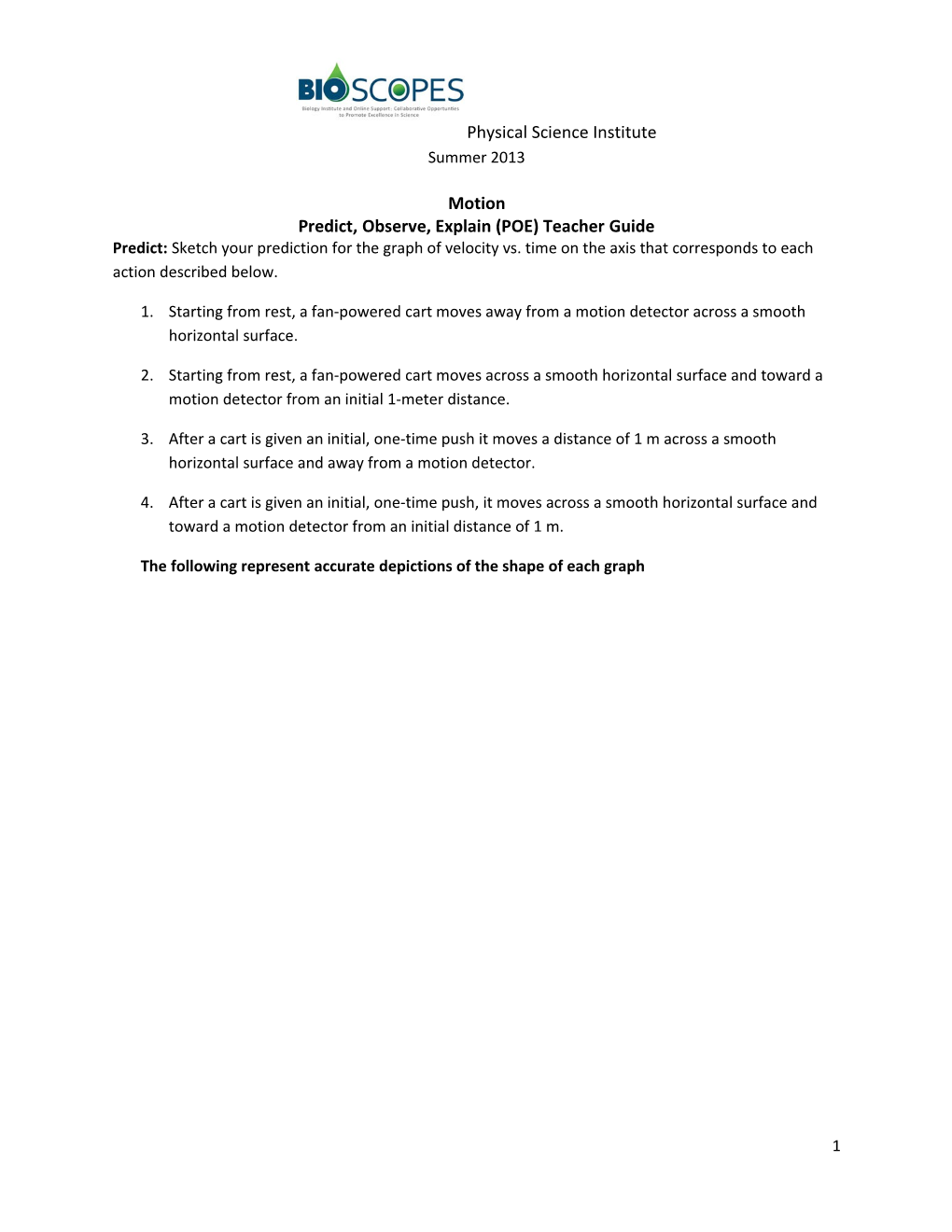# Predict, Observe, Explain (POE) Teacher GuidePhysical Science Institute

Summer 2013

Motion

Predict, Observe, Explain (POE) Teacher Guide

Predict: Sketch your prediction for the graph of velocity vs. time on the axis that corresponds to each action described below.

1. Starting from rest, a fan-powered cart moves away from a motion detector across a smooth horizontal surface.
2. Starting from rest, a fan-powered cart moves across a smooth horizontal surface and toward a motion detector from an initial 1-meter distance.
3. After a cart is given an initial,one-time push it moves a distance of 1 m across a smooth horizontal surface and away from a motion detector.
4. After a cart is given an initial, one-time push, it moves across a smooth horizontal surface and toward a motion detector from an initial distance of 1 m.

The following represent accurate depictions of the shape of each graph

Observe: On the appropriate axes, sketchthe velocity vs. time graph that you observed for each trial.

Explain: In the space below, provide a detailed explanation for your prediction for each trial, including a comparison with your predicted velocity vs. time graphs and the actual ones observed. Include a detailed interpretation of the observed graph and discuss how the graph relates to the actual motion that you observed. It is important to note that for each example above, the direction away from the motion detector is assumed to be the positive direction.

Trial 1.

The fan cart is speeding up at a constant rate. Since the cart is moving away from the detector as it speeds up, its acceleration is positive, thus the slope of the line is positive.

Trial 2.

Since in this trial the fan powers the cart back toward the motion detector, the direction of motion is negative. Since the cart is speeding up in the negative direction, the slope of the velocity-time graph (acceleration) is negative

Trial 3.

In this trial the cart is moving due to the initial push it received at the very beginning of its motion. Since the cart continues to move across a virtually frictionless surface, it continues moving in the positive direction (away from the detector) at a constant, positive velocity.

Trial 4.

In this trial the cart is given an initial push back toward the detector. Since it moves at a constant velocityin the negative direction (toward the detector), its velocity is negative.

1